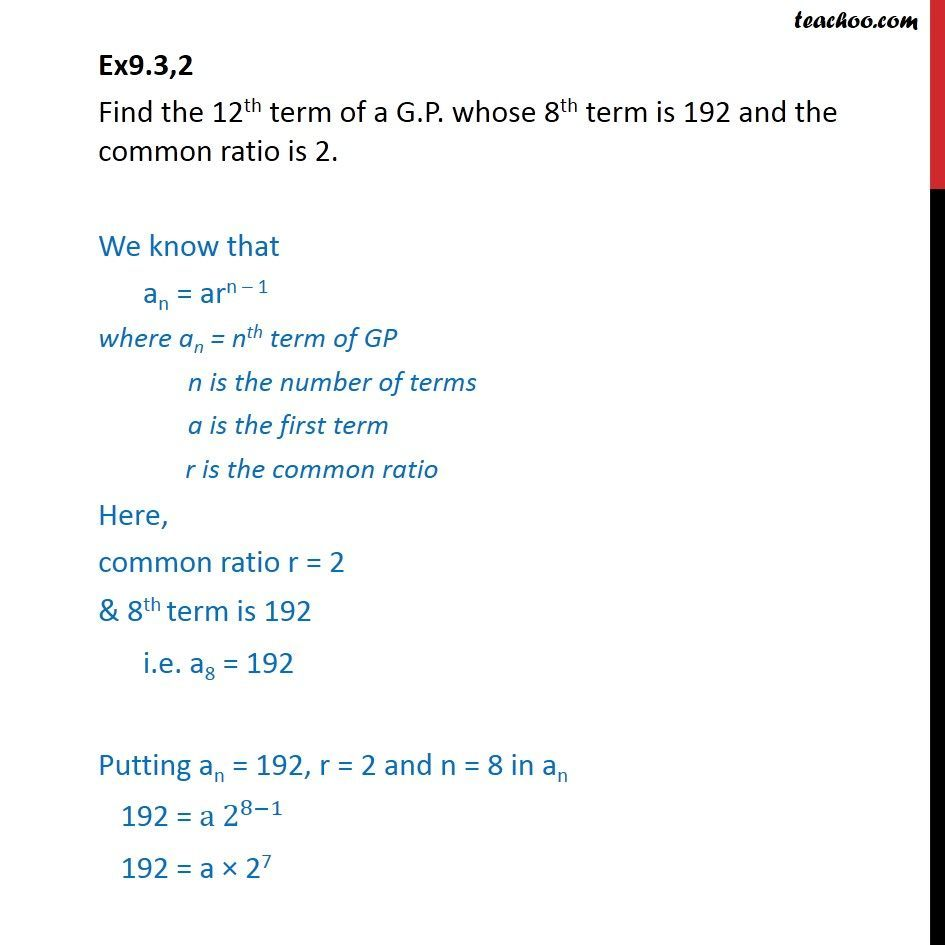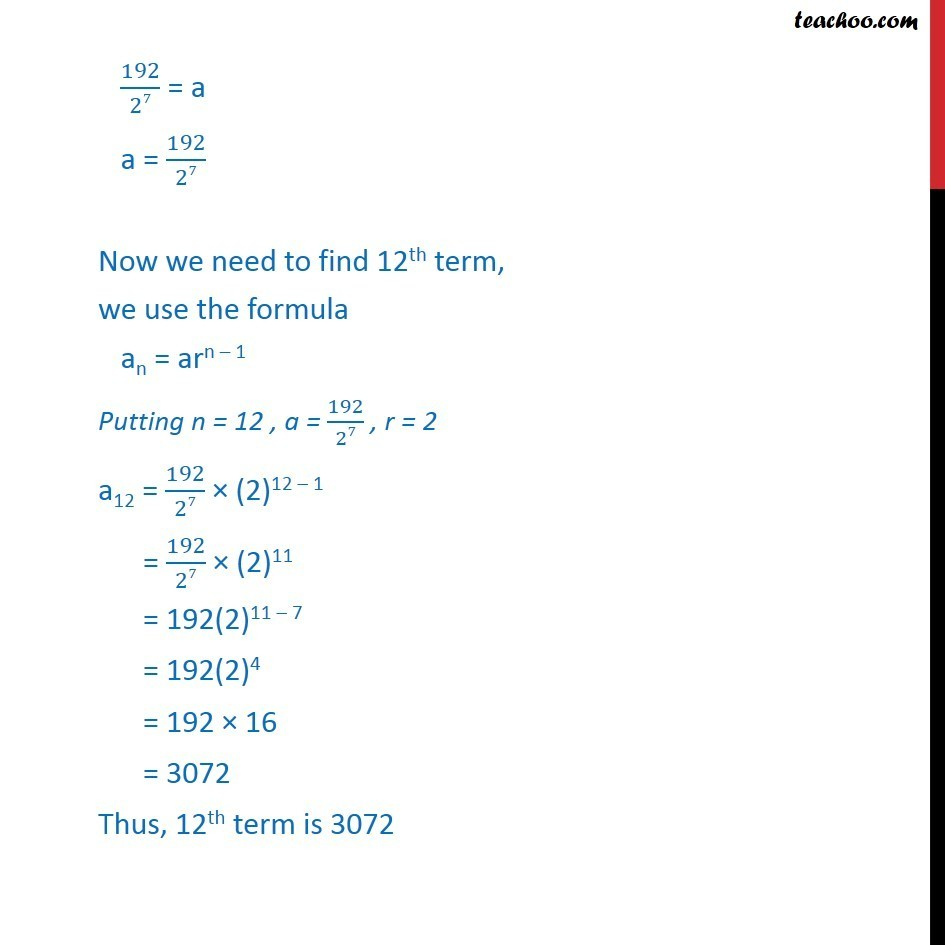Geometric Progression(GP): Formulae based

Chapter 8 Class 11 Sequences and Series
Concept wiseLearn in your speed, with individual attention - Teachoo Maths 1-on-1 Class

### Transcript

Ex9.3,2 Find the 12th term of a G.P. whose 8th term is 192 and the common ratio is 2. We know that an = arn 1 where an = nth term of GP n is the number of terms a is the first term r is the common ratio Here, common ratio r = 2 & 8th term is 192 i.e. a8 = 192 Putting an = 192, r = 2 and n = 8 in an 192 = a 2^(8 1) 192 = a 27 192/27 = a a = 192/27 Now we need to find 12th term, we use the formula an = arn 1 Putting n = 12 , a = 192/27 , r = 2 a12 = 192/27 (2)12 1 = 192/27 (2)11 = 192(2)11 7 = 192(2)4 = 192 16 = 3072 Thus, 12th term is 3072